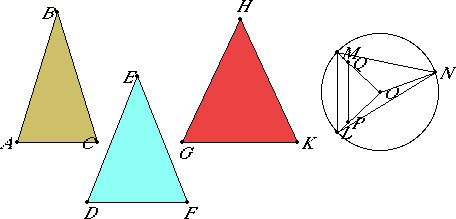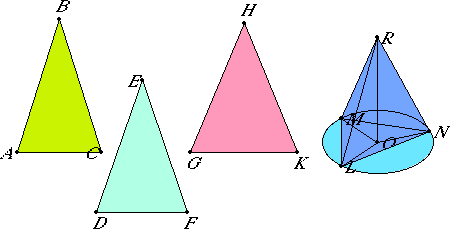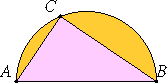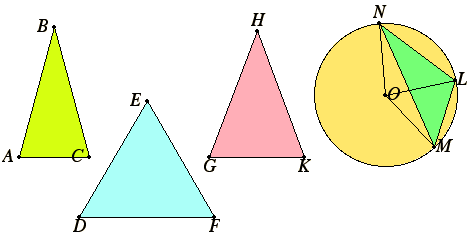# Proposition 23

To construct a solid angles out of three plane angles such that the sum of any two is greater than the remaining one: thus the sum of the three angles must be less than four right angles.

Let the angles ABC, DEF, and GHK be the three given plane angles, and let the sum of any two of them be greater than the remaining one, and further, let the sum of all three be less than four right angles.

It is required to construct a solid angle out of angles equal to the angles ABC, DEF, and GHK.

I.3

Cut off AB, BC, DE, EF, GH, and HK equal to one another, and join AC, DF, and GK.

XI.22

It is therefore possible to construct a triangle out of straight lines equal to AC, DF, and GK. Construct LMN so that AC equals LM, DF equals MN, and GK equals NL.Describe the circle LMN about the triangle LMN, and take its center O. Join LO, MO, and NO.

I say that AB is greater than LO.

For, if not, AB either equals LO, or is less.

I.8

First, let it be equal. Then, since AB equals LO, while AB equals BC, and LO equals OM, therefore the two sides AB and BC equal the two sides LO and OM respectively. And, by hypothesis, the base AC equals the base LM, therefore the angle ABC equals the angle LOM.

For the same reason the angle DEF also equals the angle MON, and the angle GHK equals the angle NOL. Therefore the sum of the three angles ABC, DEF, and GHK equals the sum of the three angles LOM, MON, and NOL.

But the sum of the three angles LOM, MON, and NOL equals four right angles, therefore the sum of the three angles ABC, DEF, and GHK equals four right angles.

But the sum is also, by hypothesis, less than four right angles, which is absurd. Therefore AB is not equal to LO.

I say next that neither is AB less than LO.

For, if possible, let it be so. Make OP equal to AB, and OQ equal to BC, and join PQ.

I.3

Then, since AB equals BC, therefore OP also equals OQ, so that the remainder LP equals QM.

Therefore LM is parallel to PQ, and LMO is equiangular with PQO.

Therefore, OL is to LM as OP is to PQ, and alternately, LO is to OP as LM is to PQ.

But LO is greater than OP, therefore LM is greater than PQ. And LM equals AC, therefore AC is greater than PQ.

Since, then, the two sides AB and BC equal the two sides PO and OQ, and the base AC is greater than the base PQ, therefore the angle ABC is greater than the angle POQ.

I.25

Similarly we can prove that the angle DEF is also greater than the angle MON, and the angle GHK is greater than the angle NOL.

Therefore the sum of the three angles ABC, DEF, and GHK is greater than the sum of the three angles LOM, MON, and NOL. But, by hypothesis, the sum of the angles ABC, DEF, and GHK is less than four right angles, therefore the sum of the angles LOM, MON, and NOL is much less than four right angles. But the sum also equals four right angles, which is absurd. Therefore AB is not less than LO.

And it was proved that neither is it equal, therefore AB is greater than LO.

XI.12
Lemma below

Next set up OR from the point O at right angles to the plane of the circle LMN so that the square on OR equals the square on AB minus the square on LO. Join RL, RM, and RN.Then, since RO is at right angles to the plane of the circle LMN, therefore RO is also at right angles to each of the straight lines LO, MO, and NO. And, since LO equals OM, and OR is common and at right angles, therefore the base RL equals the base RM.

For the same reason RN also equals each of the straight lines RL and RM. Therefore the three straight lines RL, RM, and RN equal one another.

Next, since by hypothesis the square on OR equals equals the square on AB minus the square on LO, therefore the square on AB equals the sum of the squares on LO and OR.

I.47

But the square on LR equals the sum of the squares on LO and OR, for the angle LOR is right, therefore the square on AB equals the square on RL. Therefore AB equals RL.

But each of the straight lines BC, DE, EF, GH, and HK equals AB, while each of the straight lines RM and RN equals RL, therefore each of the straight lines AB, BC, DE, EF, GH, and HK equals each of the straight lines RL, RM, and RN.

I.8

Since the two sides LR and RM equal the two sides AB and BC, and, by hypothesis, the base LM equals the base AC, therefore the angle LRM equals the angle ABC. For the same reason the angle MRN equals the angle DEF, and the angle LRN equals the angle GHK.

Therefore, out of the three plane angles LRM, MRN, and LRN, which equal the three given angles ABC, DEF, and GHK, the solid angle at R has been constructed, which is contained by the angles LRM, MRN, and LRN.

Q.E.F.

# Lemma

But how it is possible to take the square on OR equal to the square on AB minus the square on LO we can show as follows.Set out the straight lines AB and LO, and let AB be the greater. Describe the semicircle ABC on AB. Fit AC into the semicircle ABC equal to the straight line LO, not being greater than the diameter AB. Join CB.

IV.1

Since the angle ACB is an angle in the semicircle ACB, therefore the angle ACB is right.

III.31

Therefore the square on AB equals the sum of the squares on AC and CB.

I.47

Hence the square on AB equals the square on AC minus the square on CB. But AC equals LO. Therefore the square on AB equals the square on LO minus the square on CB. Therefore if we cut off OR equal to BC, then the square on AB will equal the square on LO minus the square on OR.

Q.E.F.

## Guide

This proposition shows that the necessary conditions for constructing a solid angle found in XI.20 (the sum any two angles must be less than the third) and XI.21 (the sum of the three angles must be less than four right angles) are, in fact, sufficient. It is interesting to see how parts of the construction disappear as the angles B, E, and H grow so that these conditions fail.

This proposition completes the introductory portion of Book XI. Most of the remainder deals with parallelepipedal solids and their properties.